## Time delayStarted by 4 years ago14 replieslatest reply 4 years ago429 views

My idea:

I have two input separate signals from 2 microphones placed a few millimeters apart. The signal from each microphone will be passed through an IIR filter (IIR filter as opposed to FIR filter, because i'm going to implement this in Assembly code with limited computational resources). I want to apply filter co-efficients such that there is a similar magnitude response from each line w.r.t the other line, but a constant time delay (due to the varying phase difference across the 2 lines) across them.

My questions:

1. For example, lets say i want to apply a time delay of 20 micro seconds in the second line using the IIR filter. I'd also like to have a passband as flat as possible ( from 500 Hz - 8000 Hz).

i'm not sure of the math behind this. So how can i calculate the coefficients to implement something like this ?
Thank you for any help.

[ - ]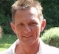Some hints ...

1. IIR-filters are not "really" computationally cheaper than FIR-filters. They are different.
Typically IIR filters cost more word-length during calculation, and might be difficult to handle without floating point numbers.
FIR filters cost more storage, but reduce mathematical operations to simpler structures and less word length is necessary.

2. Because of the feedback structure IIR filters have frequency-dependent delay.
FIR-filters instead (as long as they are used in the straight-forward manner without feedback) provide a constant time-delay which depends mainly on the tap counts.
Which is probably what you want.

3. A low pass filter at 8000 will not be difficult to design and implement.
But take care when designing the high-pass filter. As IIR-filter these filters might overshoot (requires enough headroom/word length), and they tend to get instable which might cause them to oscillate or fail completely, if not properly designed and/or if the order increases. (Second-order-section is what you should understand)
You will not find such effects with FIR-filters.

4. I'd propose you start with a simulator/calculation software like scilab or octave. (Or, if you're familiar with python: try scipy)
These programs will help you to understand the mechanisms and even you can make them calculate the coefficients.
Increase the order until the filters fail. This will help you to understand what happens.

5. If the simulation result is of sufficient quality, try to reduce complexity (filter order / number of coefficients / calculation word length) as far as possible.
Only then is the time to try it out in assembly, where code changes are most expensive.

6. Good literature is available (e.g. Rick Lyon's book), even online (from  Steve Smith). Or try out wikipedia :-)

Good luck

[ - ]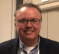Good suggestions, all.

To build on point #2... a unity-gain magnitude wideband 20ms delay is best implemented (digitally, of course) as a simple delay line buffer.  For a high side frequency of 8KHz, it wont be particularly short but it won't be particularly long, either (~500taps@fs=24KHz).

Since simplicity seems to be key and the OP needs the same simple filter dynamic on each channel - and since a mic needs a preamp and an antialiasing filter - I'd suggest implementing any passband filtering in analog/SC-filter hardware prior to sampling.

Two channels of 500-8000Hz passband isn't a complicated filter in hardware and - being identical across the 2-channels - allows the relative 20ms delay to be maintained without issue.  The digital portion then becomes a passthrough on one channel and a delay line buffer on the other.  It doesn't get easier than that!

I also agree that simulation is important.  The Scilab/Octave suggestion is great.  If implemented in the simple interpretion above, a basic WAV file processor like Goldwave would work, too.

OP: If below 500Hz needs to be preserved in some way or something else like that, please expand on the description.

______________

Not really.  Sample at 96KHz and have a 2-tap delay on one channel.

IMHO, the filtering question basically boils down to how much relative gain and phase error the OP can live with.  The suggestion of implementing the relative delay as a delay line is rooted in the assumption that absolutely no gain or phase distortion is acceptible.

If that is not the case, then other options abound.

[ - ]@bholzmayer

1. i have a 24 bit fixed point CSR chip on which i want to implement this. Based on my understanding, IIR can be implemented with fewer taps  than FIR filters.

2. Based on what i have read so far, it seems apparent that FIRs have a far greater flat frequency response. But due to point 1 i have been sticking to IIRs.

3. I was thinking of a second order IIR filter. I'll also check FIR filters.

4/5. i dont have Scilab. but i do have MATLAB. so im going to experiment and learn.

6. i am currently reading Steven's smith's book.

Thank you.

[ - ]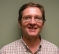Hello,

First off if you are doing audio processing then the IIR filters are absolutely the way to go.  The ear is not sensitive to phase delay.  However, you will get time domain artifacts from FIR filters such as "pre-ringing" that the ear is very sensitive to.  Again, I'm assuming you are not doing digital communications.  Just simulate with impulses, step functions and frequency sweeps with your actual code to show that the stability and performance is what you expect.

For the 20 us of delay is it possible have a sample delay buffer?  There are ways of introducing delay with all-pass networks but it is going to take some work to get there and a lot of computation.  Modern DRAM is cheap and very power efficient.  Just saying.

Have fun,

Mark Napier

[ - ]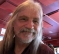So my understanding is that you want a fractional-sample delay, where you specify in advance how much delay you want.

1. If the delay is a fraction of a sample less than one sample, the only FIR way to do it might be linear interpolation.  But the magnitude of the frequency response is not so flat, particularly as you get to 1/2 sample delay.

2. An IIR way to approximate a fractional sample delay (with fractional delay between 0 and 1 sample) is with a 1st-order All-Pass Filter (APF).  The transfer function is

H(z) = (-p + z^-1) / (1 - p z^-1)

p is the pole value of the 1st-order filter and to be stable -1 < p < +1.  But for fractional sample delay, you want p < 0.  The group delay and the phase delay is not constant, but approximately constant with frequency.  The phase delay, as a function of normalized angular frequency, w, and in sample units is:

tau(w) = (1/w) arctan( ( (1-p^2)sin(w) ) / ( (1+p^2)cos(w) - 2p) )

At a frequency of 0 (DC), the group delay and phase delay is the same number and that delay, as a function of the pole p is:

tau(0) = (1+p)/(1-p)

and solving for the pole p:

p = (tau-1)/(tau+1)

note that -1 < p < 0.

3. Now to do this with **precision** delay and a flat magnitude response, you need to do this with a "polyphase interpolation" FIR but that will give you a delay that is several samples (about half of the FIR length) plus the fractional delay.  However, you can also delay the other line by the known several samples so that the delay difference is always a fraction of a sample.  Polyphase FIR filtering is a bigger topic.  But if you understand the Nyquist/Shannon sampling and reconstruction and sinc() functions, it's not hard to understand polyphase FIR filtering.

[ - ]@rbj . I like this idea of an All Pass IIR filter. But you said

"Now to do this with **precision** delay and a flat magnitude response, you need to do this with a "polyphase interpolation" FIR"

Does this mean, i have to have in series an all pass IIR filter and a polyphase interpolation FIR filter. ?

[ - ]no.  it's an alternative way of doing it.  using polyphase FIR, you have coefficients for an FIR filter for every fractional delay you want.

Let's say your FIR length is 16 samples.  so your FIR taps go from h to h.  one set of FIR coefficients will have a delay of 7.000 samples.  another set of FIR coefficients will have a delay of 7.125 samples.  another set of coefficients will give you 7.250 sample delay.  eventually you will have a set for 7.875 sample delay and one set for 8.000 samples.

you can design these coefficient sets using MATLAB to design a low-pass filter.  either a windowed-sinc function or use firpm() or firls().

[ - ]IIR allpass filters wold help here in general.

for example, if your sampling rate is 16k, then your delay is less an sample and you need a fractional delay. http://users.spa.aalto.fi/vpv/publications/icassp00-fd-slides.pdf may give you enough math background.

you skip math and just take one octave code like https://ccrma.stanford.edu/~jos/Interpolation/Matlab_Code_Lagrange_Fractional.html and try in with your code.

[ - ]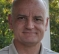I agree with the general principles espoused in the other comments here, but I think that things can be made less complicated.

However, there is an important question we need to ask first.

1) Your band of interest is 500-8000 implying sampling > 16kHZ.  If you are sampling at 48 kHz (very common) then one sample delay is approximately the 20 usec you want.  What is the sampling rate you have chosen, or what is the rate available to you?

2) If the sampling rate does not allow for integer sample delays to achieve the desired delay, then I propose a simple interpolation filter on one of the channels to form the delay, and a simple delay on the other to match the integer part of the delay in the interpolation filter.

a) There are multiple ways to create the interpolation filter.  Farrow interpolation, FIR interpolation filter, linear interpolation(not recommended), splines, Bessel IIR, etc.

3) After the delay is applied to each channel, the exact same filter can be used for the bandwidth control down to 8000.

4) If your processor is a 32 bit engine, then I believe that IIR filters will be OK.  I have a rule of thumb for IIR filters related to the internal feedback variables within the biquads. They will usually grow by a factor of 1/(1-r) compared to the data, where r is the radius of the poles for that biquad.  Sometimes the poles can be very close to the unit circle, so this gain can get quite large.  If you are using 16 bit data, then the headroom for 32 bit variables should be OK for most cases.

My 2 cents

David

[ - ]I apologize for the long delay to reply.  To answer the questions asked in the replies, I am trying to implement the idea from this paper titled "Digital Filter Array Optimization for Directivity Pattern ".

1. My sampling frequency, similar to the paper, is 16 kHz.

2.  My processor is a CSR chip, similar to the paper, a 24 bit fixed point engine.

I can't figure out how the Author of the paper calculated the filter co-efficients.

p:s - i have begun reading the book. Steven Smith' The Scientist & Engineer's Guide to Digital SIgnal processing. I again apologize for the delay in reply. Had some personal issue to attend to. Thank you all your replies.

[ - ]From the paper, I assume that you do NOT want to compensate for the delay between the microphone signals but instead want to preserve the delay (?) because it contains the direction information.
Or, do you want to shift the delay until you find a value which gives you the direction information?

And why, if (assuming from the paper) the output has to do with human hearing, are you only interested in frequencies >500Hz?

It might help to get some more helpful propositions, if you would share what you're really trying to achieve ...
because it's something very different if you want to calculate the delay or compensate it or preserve it - and you might want to go different paths for each.

[ - ]Yes. you are right. i do not want to compensate for the delay. But rather impose a delay. I'll expand further and say that I want to implement different cardioid patterns using 2 microphones just like shown in this document below.

The gist of the idea is .. if I have 2 microphones placed in an axis with a distance X between them, then using a formula for time delay, (with a Beta value of 1 for a cardioid response) the time delay amounts to 26.17 useconds. Since there are two mic input lines, if i can delay the second mic line by 26.17 us (wrt to the first mic line), i can implement a cardioid pattern as shown in the document above.

While researching this idea, i found the paper ("Digital Filter Array Optimization for Directivity Pattern ".) i posted earlier. I want to understand how to cause a delay in the second microphone to be able to achieve this cardioid pattern.

I realize that using a IIR filter I can implement this. Based on my limited understanding of filters ( and with access to MATLAB), i can obtain coefficients to implement HP/LP/Notch .. etc. but what I don't know how to do is

1. What will be the time delay for a said filter? How do I calculate this?

I have 2 objectives

Short term objective: Find out how to calculate time delay for a given filter in relation to the above objective.

Long term objective:

How does one go about designing a filter? Based on all your replies, given a problem, all of you seem to know instantaneously what filter (FIR/IIR or otherwise) to choose and how many taps it should have and the effect of phase and magnitude this filter will have on a signal.

b. Do you start with plotting poles and zeros on an unit circle?

I dont seem to know how to approach a given problem while designing filters. so any guidance would be very much appreciated.

I would like to know how to do this as well.

[ - ]You already had plenty on how to design filters. I will add some notes on delay. filter delay is both constant integer (actual delay caused by registers) and group delay.

group delay is -diff of phase with respect to frequency but don't worry, use matlab function grpdelay(b,a). It gives delay in samples against frequency axis.

For iir you will notice it is not linear but for symmetric fir it is fixed constant. Thus for iir it depends on frequency.

[ - ]I'm very unfamiliar with all this cardioid things.
But if I had to design an IIR-bandpass-filter, I'd probably start with a bode diagram and butterworth filters, because they are very easy to imagine and very straigthforward to calculate. (Check wikipedia or electronic books)

• minimum required stop band (lower end) attenuation: let's say -60dB
• minimum required stop band (higher end): let's say -60dB
• maximum tolerable passband level change: let's say 3dB
• lower edge (high-pass):  let's say 500Hz
• higher edge (low-pass): let's say 4kHz
• define necessary sample rate: let's say 16kHz

Most important: before trying to find a realization, try to reduce the requirements as much as possible, because at that point you're not so much distracted of implementation aspects ;-)

Now, I'd enter these values into a filter design software.
You might use the Matlab tool (fvtool or so).
But I'd recommend ScopeIIR from https://iowegian.com.
You can download and use it to play around with it at no cost.
Only if you want to get out the coefficient results, you need the licensed version. But I mean, it's a great tool :-)

In less than 5 minutes, you'll see amplitude and phase response.
Play around with the sample rate, filter order, cutoff frequencies until you understand the possibilities of this filter.

Then change the filter type, and in another ten minutes, you've probably found out if this filter is good for your purpose.